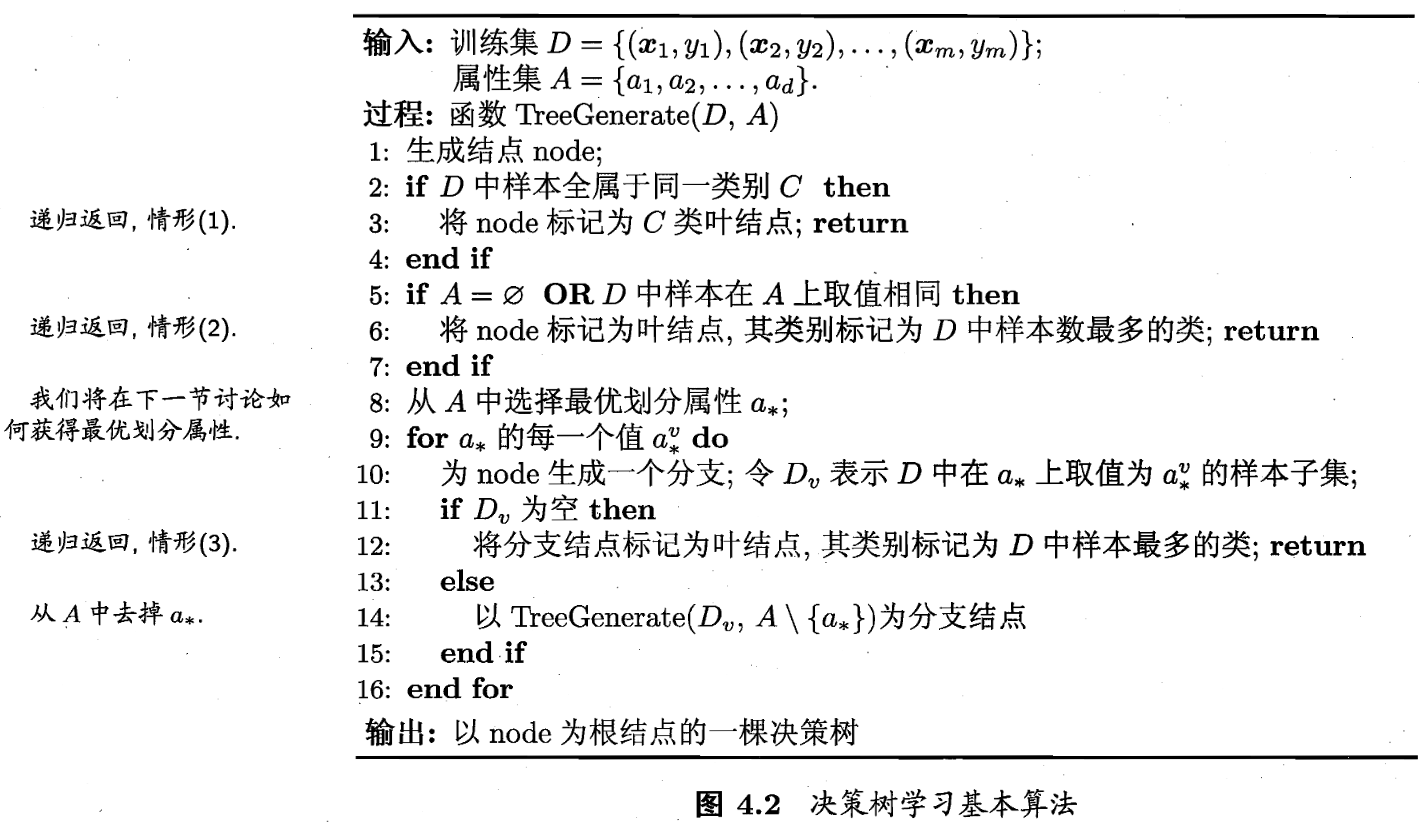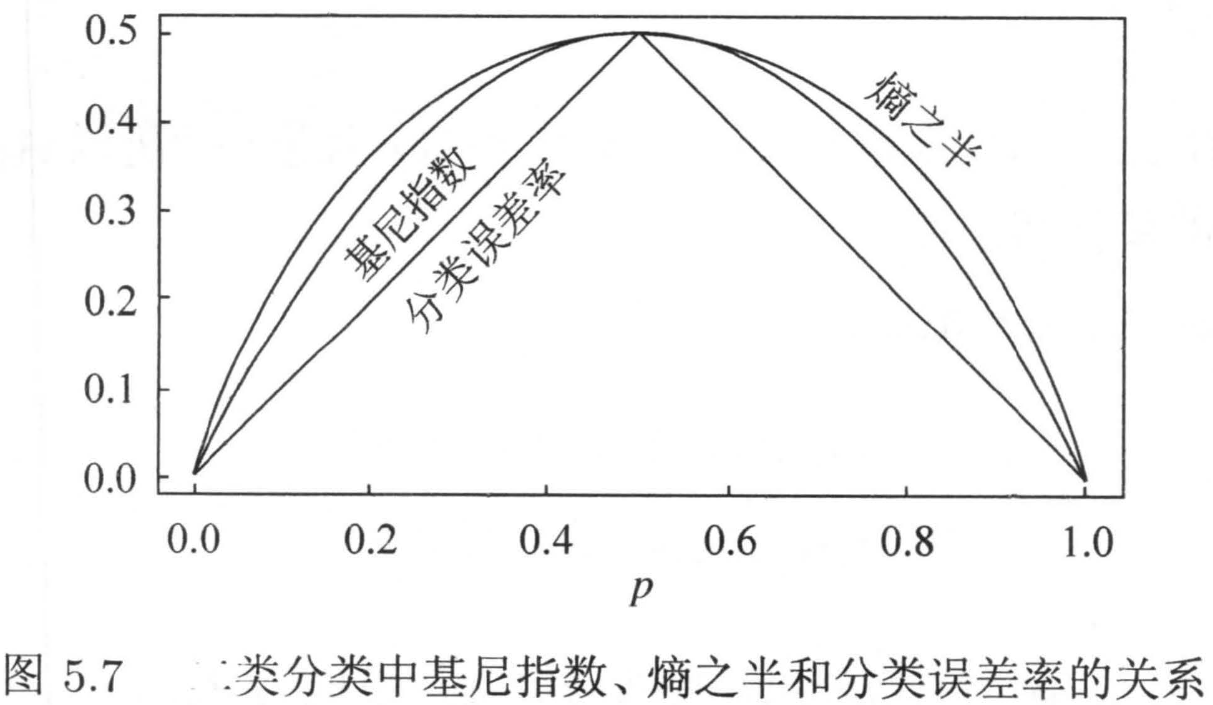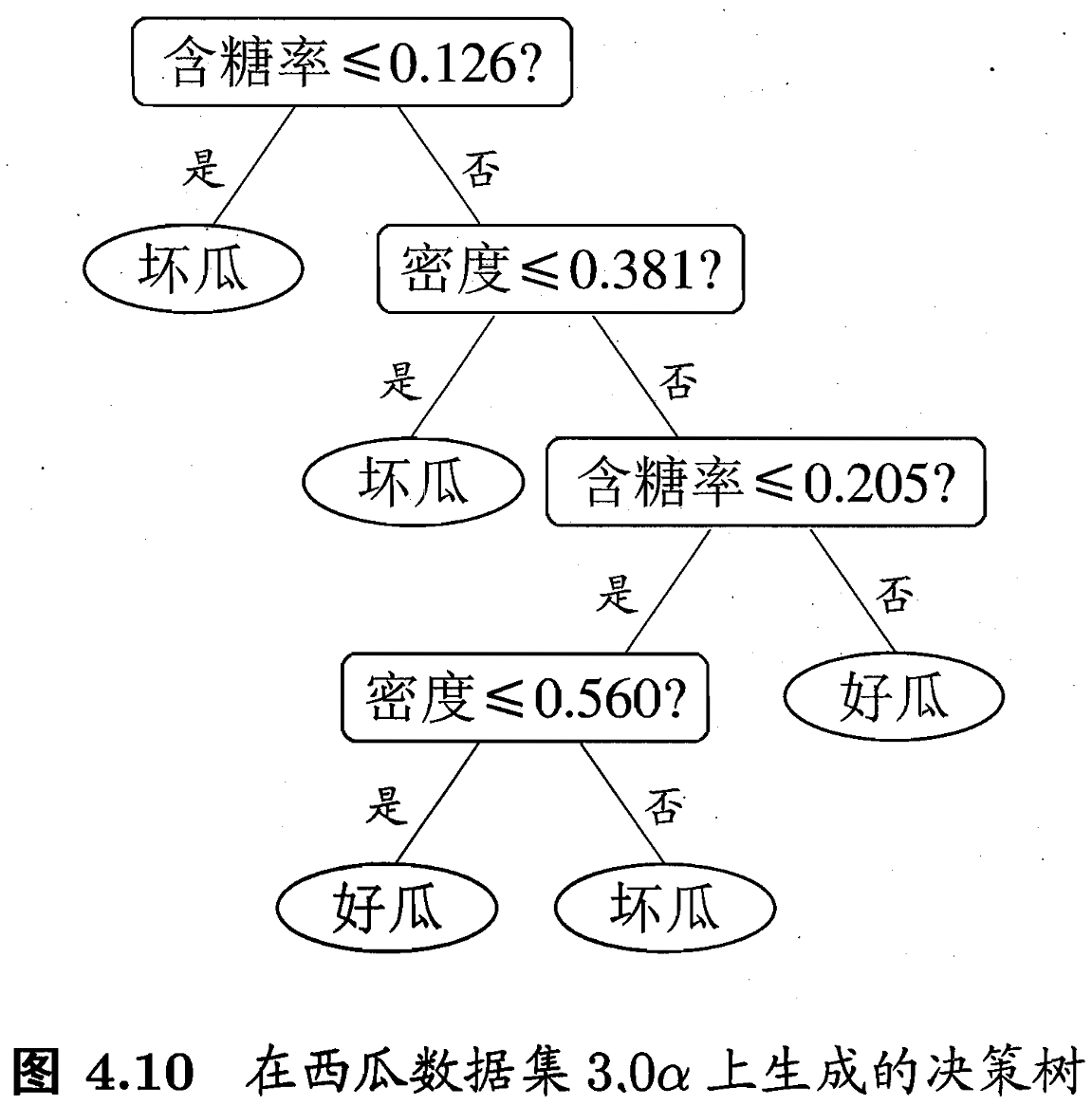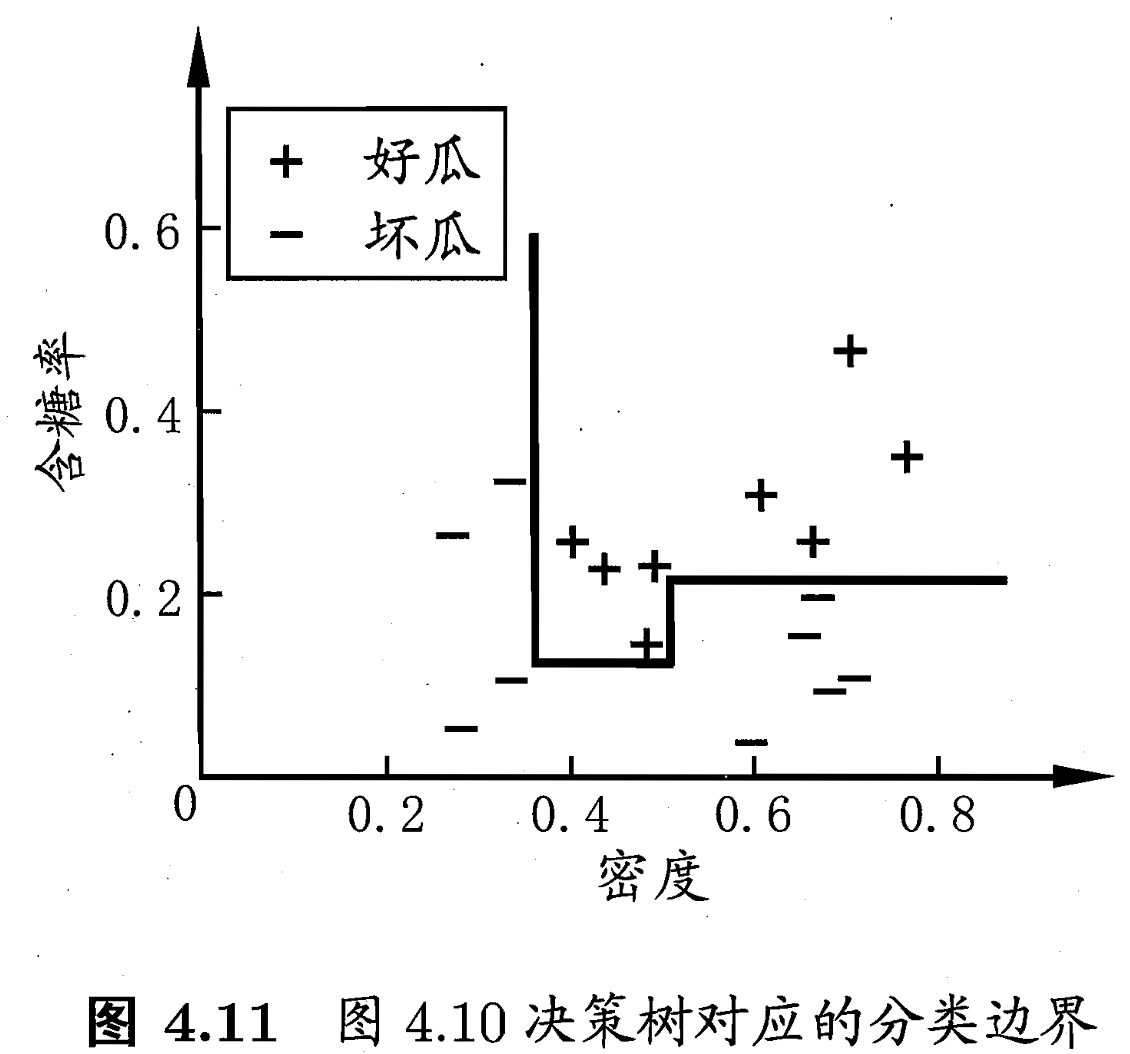# 机器学习笔记-决策树

## 决策树的基本流程## 决策树特征选择

### 信息增益比

C4.5（1993年提出）算法是使用“信息增益比”来选择最优划分属性的。需注意的是，信息增益比准则对可取值数目较少的属性有所偏好。因此，实际C4.5算法并不是直接选择增益比最大的候选划分属性，而是使用了一个启发式：先从侯选划分属性中找出信息增益高于平均水平的属性，再从中选择增益比最高的。

### 基尼指数

“基尼指数”（Gini index）也是一个较常用的特征选择策略，CART（Classification and Regression Tree）决策树就是应用”基尼指数”来做特征选择的。## CART树

CART树（classification and regression tree, CART）在1984年提出，是经典的决策树学习方法。CART树既可以用于分类也可以用于回归。

CART假设决策树是二叉树，内部结点特征的取值为“是”和“否”，左分支是取值是“是”的分支，右分支是取为“否”的分支。这样的决策树等价于递归地二分每个特征，将输入空间即特征空间划分为有限个单元，并在这些单元上确定预测概率分布。CART树由决策树的生成后剪枝组成，其中决策树的生成包含特征选择的策略。

### CART树生成

#### 回归树的生成

$f(x) = \sum_{m=1}^{M} c_m \cdot I(x \in R_m)$ ，当输入空间的划分确定时，可以用平方误差 $\sum_{X_i \in R_m} (y_i - f(x_i))^2$ 来表示回归树对于训练数据的预测误差，用平方误差最小的准则求解每个单元上的最优输出值。易知，单元 $R_m$ 上的 $c_m$ 的最优值 $\overline{c}_m$$R_m$ 上的所有输入实例 $x_i$ 对应的输出 $y_i$ 的均值，即 $\overline{c}_m = ave(y_i|x_i \in R_m)$

$min_{j,s} \left [ min_{c_1} \sum_{x_i \in R_1(j,s) } (y_i - c_1)^2 + min_{c_2} \sum_{x_i \in R_2(j,s)} (y_i - c_2)^2 \right ]$

（1）选择最优切分变量 $j$ 扫描切分点 $s$ 。具体地，求解下式，遍历变量 $j$ ，对固定的切分变量 $j$ 扫描切分点 $s$ ，选择使下式达到最小值的 $(j,s)$

$min_{j,s} \left [ min_{c_1} \sum_{x_i \in R_1(j,s) } (y_i - c_1)^2 + min_{c_2} \sum_{x_i \in R_2(j,s)} (y_i - c_2)^2 \right ]$

（2）用选定的对 $(j,s)$ 划分区域并决定相应的输出值：

$R_1(j,s) = \{ x|x^{(j)} \leqslant s \}$$R_2(j,s) = \{ x|x^{(j)} > s \}$

$\overline{c}_m = \frac{1}{N_m} \sum_{x_i \in R_m(j,s)} y_i \ , \ \ x \in R_m \ , m = 1,2$

（3）继续对两个子区域调用步骤（1），（2），直至满足停止条件。

（4）将输入空间划分为 $M$ 个区域 $R_1, R_2, ..., R_M$ ，生成决策树： $f(x) = \sum_{m=1}^{m} \overline{c}_m \cdot I(x \in R_m)$

#### 分类树的生成

（1）设结点的训练数据集为 $D$ ，计算现有特征对该数据集的基尼指数。此时，对每一个特征 $A$ ，对其可能取的每个值 $a$ ，根据样本点对 $A=a$ 的测试为“是”或“否”将 $D$ 分割成 $D_1$$D_2$ 两部分，计算 $A=a$ 的基尼指数。

（2）在所有可能的特征 $A$ 以及它们所有可能的切分点 $a$ 中，选择基尼指数最小的特征及其对应的切分点 $a$ 中，选择基尼指数最小的特征及其对应的切分点作为最优特征与最优切分点。依最优特征与最优切分点，从现结点生成两个子结点，将训练数据集依特征分配到两个子结点中去。

（3）对两个子结点递归地调用（1），（2），直至满足停止条件（停止条件是结点中的样本个数小于预定阈值、或样本集的基尼指数小于预定阈值、或样本基本属于同一类、或无更多特征）。

（4）生成CART决策树。

### CART树后剪枝

CART剪枝算法从“完全生长”的决策树的底端剪去一些子树，使决策树变小（模型变简单），从而能够对未知数据有更准确的预测。CART剪枝算法由两步组成：首先从生成算法产生的决策树 $T_0$ 底端开始不断剪枝，直到 $T_0$ 的根结点，形成一个子树序列 $\{T_0,T_1,...,T_n\}$ ；然后通过交叉验证在独立的验证数据集上对子树序列进行测试，从中选择最优子树。

#### CART剪枝过程叙述

1.剪枝，形成一个子树序列

$t$ 为根结点的子树 $T_t$ 的损失函数是 $C_a(T_t) = C(T_t) + a |T_t|$

$a=0$$a$ 充分小时，有不等式 $C_a(T_t) < C_a(t)$

$a$ 增大时，在某一 $a$$C_a(T_t) = C_a(t)$

$a$ 再增大时，不等式则会反向。只要 $a=\frac{C(t) - C(T_t)}{|T_t| - 1}$$T_t$$t$ 有相同的损失函数值，而 $t$ 的结点少，因此 $t$$T_t$ 更可取，对 $T_t$ 进行剪枝。

2.在剪枝得到的子树序列 $T_0,T_1,...,T_n$ 中通过交叉验证选取最优子树 $T_a$

#### CART剪枝算法

（1）设 $k=0,T=T_0$

（2）设 $a=+\infty$

（3）自下而上地对各内部结点 $t$ 计算 $C(T_t)$$|T_t|$ 以及 $g(t)=\frac{C(t) - C(T_t)}{|T_t| - 1}$$a=min(a,g(t))$ 。其中， $T_t$ 表示以 $t$ 为根结点的子树，$C(T_t)$ 是对训练数据的预测误差，$|T_t|$$T_t$ 的叶结点个数。

（4）对 $g(t)=a$ 的内部结点 $t$ 进行剪枝，并对叶结点 $t$ 以多数表决法决定其类，得到树 $T$

（5）设 $k=k+1, a_k=a, T_k=T$

（6）如果 $T_k$ 不是由根结点及两个叶结点构成的树，则回到步骤（2）；否则令 $T_k=T_n$

（7）采用交叉验证法在子树序列 $T_0,T_1,...,T_n$ 中选取最优子树 $T_a$

## 决策树其它要点

### 连续值处理

$T_a = \left \{ \frac{a^i+a^{i+1}}{2} \ \ | 1 \leqslant i \leqslant n-1 \right \}$

### 分类边界## 参考文献

1. 李航 《统计学习方法》第二版 P67-89
2. 周志华 《机器学习》P73-95